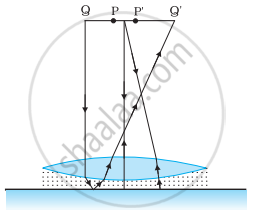# Shows an Equiconvex Lens (Of Refractive Index 1.50) in Contact with a Liquid Layer on Top of a Plane Mirror the Liquid is Removed and the Experiment is Repeated - Physics

Numerical

Figure shows an equiconvex lens (of refractive index 1.50) in contact with a liquid layer on top of a plane mirror. A small needle with its tip on the principal axis is moved along the axis until its inverted image is found at the position of the needle. The distance of the needle from the lens is measured to be 45.0 cm. The liquid is removed and the experiment is repeated. The new distance is measured to be 30.0 cm. What is the refractive index of the liquid?#### Solution

Focal length of the convex lens, f1 = 30 cm

The liquid acts as a mirror. Focal length of the liquid = f2

Focal length of the system (convex lens + liquid), f = 45 cm

For a pair of optical systems placed in contact, the equivalent focal length is given as:

1/"f" = 1/"f"_1 + 1/"f"_2

1/"f"_2 = 1/"f" - 1/"f"_1

= 1/45 - 1/30

= -1/90

∴ f2 = −90 cm

Let the refractive index of the lens be μ1 and the radius of curvature of one surface be R. Hence, the radius of curvature of the other surface is −R.

R can be obtained using the relation:

1/"f"_1 = (μ_1 - 1)(1/"R" + 1/(-"R"))

1/30 = (1.5 - 1) (2/"R")

∴ R = 30/(0.5 xx 2) = 30 cm

Let μ2 be the refractive index of the liquid.

Radius of curvature of the liquid on the side of the plane mirror = ∞

Radius of curvature of the liquid on the side of the lens, R = −30 cm

The value of μ2 can be calculated using the relation:

1/"f"_2 = (μ_2 - 1)[1/(-"R") - 1/∞]

(-1)/90 = (μ_2 - 1)[1/(+30) - 0]

μ_2 - 1 = 1/3

∴ μ2 = 4/3 = 1.33

Hence, the refractive index of the liquid is 1.33.

Concept: Refraction at Spherical Surfaces and Lenses - Refraction by a Lens
Is there an error in this question or solution?

#### APPEARS IN

NCERT Physics Part 1 and 2 Class 12
Chapter 9 Ray Optics and Optical Instruments
Exercise | Q 9.32 | Page 348
NCERT Class 12 Physics Textbook
Chapter 9 Ray Optics and Optical Instruments
Exercise | Q 38 | Page 350
Share